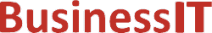O nás     Inzerce     KontaktSpolehlivé informace o IT již od roku 2011[Linux manuál]

# lgamma, lgammaf, lgammal: log gama funkce

Originální popis anglicky: lgamma, lgammaf, lgammal - log gamma function

Návod, kniha: POSIX Programmer's Manual

## STRUČNĚ

#include <math.h>

double lgamma(double x);

float lgammaf(float x);

long double lgammal(long double x);

extern int signgam;

## POPIS / INSTRUKCE

These functions shall compute

The argument x need not be a non-positive integer (Gamma(x) is defined over the reals, except the non-positive integers).
The sign of Gamma(x) is returned in the external integer signgam.
These functions need not be reentrant. A function that is not required to be reentrant is not required to be thread-safe.
An application wishing to check for error situations should set errno to zero and call feclearexcept(FE_ALL_EXCEPT) before calling these functions. On return, if errno is non-zero or fetestexcept(FE_INVALID | FE_DIVBYZERO | FE_OVERFLOW | FE_UNDERFLOW) is non-zero, an error has occurred.

## NÁVRATOVÁ HODNOTA

Upon successful completion, these functions shall return the logarithmic gamma of x.
If x is a non-positive integer, a pole error shall occur and lgamma(), lgammaf(), and lgammal() shall return +HUGE_VAL, +HUGE_VALF, and +HUGE_VALL, respectively.
If the correct value would cause overflow, a range error shall occur and lgamma(), lgammaf(), and lgammal() shall return ±HUGE_VAL, ±HUGE_VALF, and ±HUGE_VALL (having the same sign as the correct value), respectively.
If x is NaN, a NaN shall be returned.
If x is 1 or 2, +0 shall be returned.
If x is ±Inf, +Inf shall be returned.

## CHYBY / ERRORY

These functions shall fail if:
Pole Error
The x argument is a negative integer or zero.
If the integer expression (math_errhandling & MATH_ERRNO) is non-zero, then errno shall be set to [ERANGE]. If the integer expression (math_errhandling & MATH_ERREXCEPT) is non-zero, then the divide-by-zero floating-point exception shall be raised.
Range Error
The result overflows.
If the integer expression (math_errhandling & MATH_ERRNO) is non-zero, then errno shall be set to [ERANGE]. If the integer expression (math_errhandling & MATH_ERREXCEPT) is non-zero, then the overflow floating-point exception shall be raised.

The following sections are informative.

None.

## APPLICATION USAGE

On error, the expressions (math_errhandling & MATH_ERRNO) and (math_errhandling & MATH_ERREXCEPT) are independent of each other, but at least one of them must be non-zero.

None.

None.

## SOUVISEJÍCÍ

exp() , feclearexcept() , fetestexcept() , isnan() , the Base Definitions volume of IEEE Std 1003.1-2001, Section 4.18, Treatment of Error Conditions for Mathematical Functions, <math.h> Portions of this text are reprinted and reproduced in electronic form from IEEE Std 1003.1, 2003 Edition, Standard for Information Technology -- Portable Operating System Interface (POSIX), The Open Group Base Specifications Issue 6, Copyright (C) 2001-2003 by the Institute of Electrical and Electronics Engineers, Inc and The Open Group. In the event of any discrepancy between this version and the original IEEE and The Open Group Standard, the original IEEE and The Open Group Standard is the referee document. The original Standard can be obtained online at http://www.opengroup.org/unix/online.html .
 2003 IEEE/The Open Group
©2011-2020 BusinessIT.cz, ISSN 1805-0522 | Názvy použité v textech mohou být ochrannými známkami příslušných vlastníků.
Provozovatel: Bispiral, s.r.o., kontakt: BusinessIT(at)Bispiral.com | Inzerce: Best Online Media, s.r.o., zuzana@online-media.cz
O vydavateli | Pravidla webu BusinessIT.cz a ochrana soukromí | pg(9268)